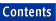Apache C++ Standard Library Reference Guide

## upper_bound()

Library:  Algorithms

`Function`

No Entries

### Summary

An algorithm that determines the last valid position for a value in a sorted container

### Synopsis

```#include <algorithm>

namespace std {
template <class ForwardIterator, class T>
ForwardIterator
upper_bound(ForwardIterator start, ForwardIterator finish,
const T& value);

template <class ForwardIterator, class T, class Compare>
ForwardIterator
upper_bound(ForwardIterator start, ForwardIterator finish,
const T& value, Compare comp);
}
```

### Description

The upper_bound() algorithm is one of a set of binary search algorithms. All of these algorithms perform binary searches on ordered sequences. Each algorithm has two versions. The first version uses operator<() to perform the comparison, and assumes that the sequence has been sorted using that operator. The second version allows you to include a function object of type Compare, and assumes that Compare is the function used to sort the sequence. The function object must be a binary predicate.

The upper_bound() algorithm finds the last position in a sequence that value can occupy without violating the sequence's ordering. upper_bound()'s return value is the iterator for the first element in the sequence that is greater than value, or, when the comparison operator is used, the first element that does NOT satisfy the comparison function. Because the algorithm is restricted to using the less than operator or the user-defined function to perform the search, upper_bound() returns an iterator i in the range [start, finish) such that for any iterator j in the range [start, i) the appropriate version of the following conditions holds:

!(value < *j)

or

comp(value, *j) == false

### Complexity

upper_bound() performs at most log(finish - start) + 1 comparisons.

### Example

```//
//  ul_bound.cpp
//

#include <vector>
#include <algorithm>
#include <functional>
#include <iostream>

int main ()
{
typedef std::vector<int, std::allocator<int> > Vector;
typedef Vector::const_iterator                 Iterator;

const int a[] = { 0, 1, 2, 2, 3, 4, 5, 5, 5, 6, 7 };

// Set up a vector.
const Vector v (a, a + sizeof a / sizeof *a);

// Try lower_bound variants.
Iterator it1 = std::lower_bound (v.begin (),v.end (), 3);
Iterator it2 = std::lower_bound (v.begin (),v.end (), 2,
std::less<int>());

// Try upper_bound variants.
Iterator it3 = std::upper_bound (v.begin (), v.end (), 3);
Iterator it4 = std::upper_bound (v.begin (), v.end (), 2,
std::less<int>());

std::cout << "\n\nThe upper and lower bounds of 3: ( "
<< *it1 << " , " << *it3
<< " ]\n\n\nThe upper and lower bounds of 2: ( "
<< *it2 << " , " << *it4 << " ]\n";

return 0;
}

Program Output:
```
```
The upper and lower bounds of 3: ( 3 , 4 ]

The upper and lower bounds of 2: ( 2 , 3 ]
```